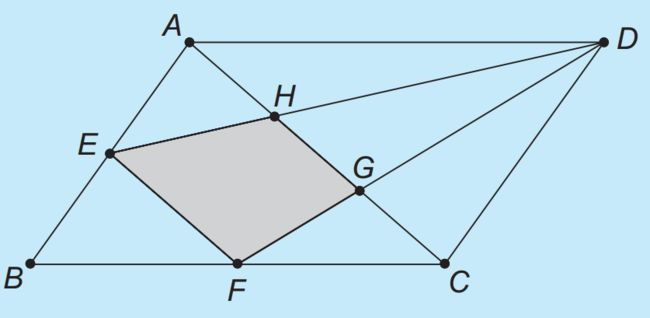# Deploying laser beams

Geometry Level 3Given a parallelogram $ABCD$ with area of $24\text{ cm}^2$ with points $E$ and $F$ as the midpoint of the lines $AB$ and $BC$ respectively. What is the area of the quadrilateral $EFGH$?

×